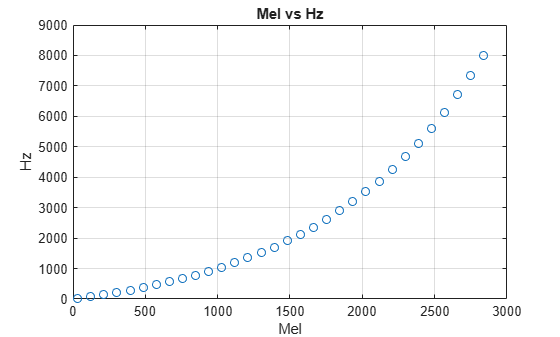# mel2hz

Convert from mel scale to hertz

## Syntax

``hz = mel2hz(mel)``

## Description

example

````hz = mel2hz(mel)` converts values on the mel frequency scale to values in hertz.```

## Examples

collapse all

Set two bounding frequencies in Hz and then convert them to the mel scale.

`b = hz2mel([20,8000]);`

Generate a row vector of 32 values uniformly spaced on the mel scale.

`melVect = linspace(b(1),b(2),32);`

Convert the row vector of values into equivalent frequencies in Hz.

`hzVect = mel2hz(melVect);`

Plot the two vectors for comparison. As mel values increase linearly, Hz values increase exponentially.

```plot(melVect,hzVect,'o') title('Mel vs Hz') xlabel('Mel') ylabel('Hz') grid on```## Input Arguments

collapse all

Input frequency on the mel scale, specified as a scalar, vector, matrix, or multidimensional array.

Data Types: `single` | `double`

## Output Arguments

collapse all

Output frequency in Hz, returned as a scalar, vector, matrix, or multidimensional array the same size as `mel`.

Data Types: `single` | `double`

## Algorithms

The frequency conversion from the mel scale to Hz uses the following formula:

`$hz=700\left({10}^{\frac{mel}{2595}}-1\right)$`

 O'Shaghnessy, Douglas. Speech Communication: Human and Machine. Reading, MA: Addison-Wesley Publishing Company, 1987.

## SupportGet trial now